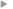## Distributional regression and its evaluation with the CRPS: Bounds and convergence of the minimax risk

#### Pic, Romain ; Dombry, Clément ; Naveau, Philippe ; Taillardat, Maxime

Année de publication
2022
Résumé
<p align=justify>The theoretical advances in the properties of scoring rules over the past decades have broadened the use of scoring rules in probabilistic forecasting. In meteorological forecasting, statistical postprocessing techniques are essential to improve the forecasts made by deterministic physical models. Numerous state-of-the-art statistical postprocessing techniques are based on distributional regression evaluated with the continuous ranked probability score (CRPS). However, the theoretical properties of such evaluations with the CRPS have solely considered the unconditional framework (i.e. without covariates) and infinite sample sizes. We extend these results and study the rate of convergence in terms of the CRPS of distributional regression methods. We find the optimal minimax rate of convergence for a given class of distributions and show that the k-nearest neighbor method and the kernel method reach this optimal minimax rate.</p>
Texte intégralAccès à la notice sur le site du portail documentaire de Météo-FranceListe complète des notices publiques